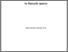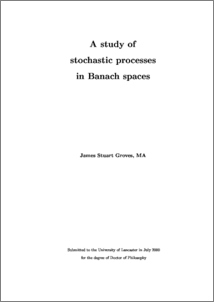# A study of stochastic processes in Banach spaces

Groves, James Stuart (2000) A study of stochastic processes in Banach spaces. PhD thesis, UNSPECIFIED.Preview
PDF (2000grovesphd)
2000grovesphd.pdf - Accepted Version

## Abstract

The theory of 2-convex norms is applied to Banach space valued random vectors. Use is made of a norm on random vectors, introduced by Pisier, equal to the 2-absolutely summing norm on an associated space of operators. For Q the variance of some centred Gaussian random vector in a separable Banach space it is shown that, necessarily, Q factors through l2 as a product of 2-summing operators. This factorisation condition is sufficient when the Banach space is of Gaussian type 2. The stochastic integral of a family of operators with respect to a cylindrical Q-Wiener process is shown to exist under a Hölder continuity condition involving the 2-summing norm. A Langevin equation dZt + ΛZtdt = dBt with values in a separable Banach space is studied. The operator Λ is closed and densely defined. A weak solution (Zt ; Bt), where Zt is centred, Gaussian and stationary while Bt is a Q-Wiener process, is given when iΛ and iΛ* generate C0 groups and the resolvent of Λ is uniformly bounded on the imaginary axis. Both Zt and Bt are stochastic integrals with respect to a spectral Q-Wiener process. The convolution of two arcsine probability densities is shown to be an elliptic integral. Ensembles (Xn)n≥1 of random Hermitian matrices are considered. Each Xn is n by n with distribution invariant under unitary conjugation and induced by a positive weight function on R. New proofs are given of results, due to Boutet de Monvel, Pastur, Shcherbina and Sodin, on the behaviour of the empirical distribution of the eigenvalues of Xn as n tends to infinity. Results in analytic function theory are proved. An H∞ interpolating sequence in the disc D whose Horowitz product does not lie in the Bergman space L2a(D) is exhibited. A condition satisfied by Banach spaces of non-trivial analytic Lusin cotype is obtained.

Item Type:
Thesis (PhD)
Departments:
ID Code:
125004
Deposited By:
Deposited On:
08 May 2018 10:06
Refereed?:
No
Published?:
Published